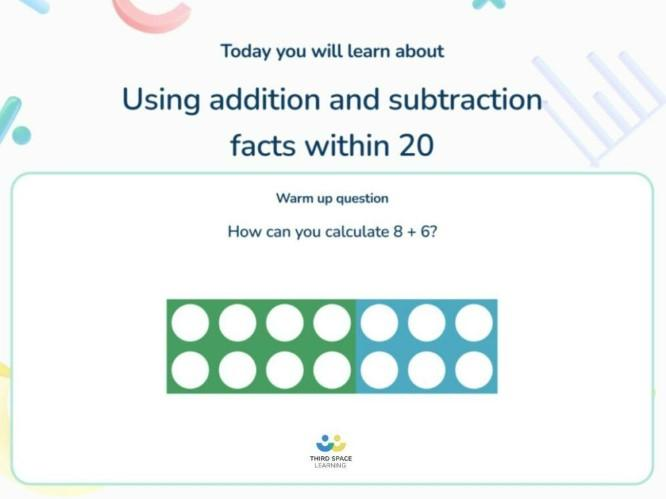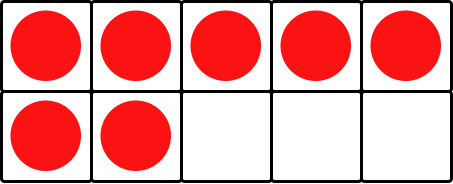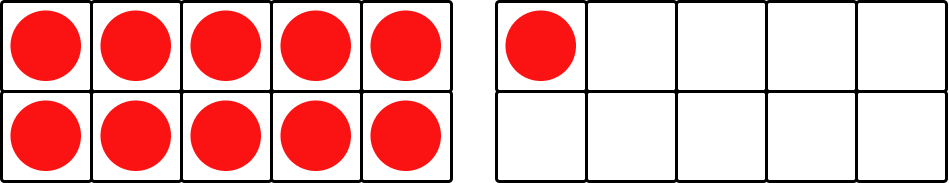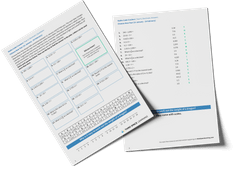Math teaching support you can trustone-on-one tutoring sessionsschools supported

[FREE] Fun Math Games & Activities Packs

Always on the lookout for fun math games and activities in the classroom? Try our ready-to-go printable packs for students to complete independently or with a partner!

# What Is A Ten Frame? Explained For Elementary School Teachers

Ten frames are becoming a popular teaching tool for use in the classroom. Often used in early grades to consolidate understanding of numbers to ten, they can also be utilized in higher grades, especially when introducing concepts related to tenths.

In this blog, we will look at what a ten frame is and think about some activities that can be used for in elementary schools.

### What is a ten frame?

A ten frame consists of a rectangular frame divided into two rows of five boxes each, creating a total of ten boxes. Each box in the ten frame represents one unit. Children can place counters or any other manipulative used for counting on it.

They are usually introduced for place value activities and number bonds to ten in the early grades of elementary school. They can also be used to help children understand the concept of a tenth in both fractions and decimals.

Our number system is a base-10 number system which means that we have 10 different numerals (0-9) which can be placed into any place value column and that each position has a value in powers of ten. For this reason, we often break numbers into groups of ten (think the metric systems of measurement).

### How does a ten frame work?

A ten frame is used to represent numbers within and up to ten. Children can compose and decompose each number in a variety of ways which helps them develop number sense and to understand different visual representations of numbers.

Usually, ten frames are used with manipulative resources but they can also be used visually on a worksheet or using a laminated frame and dry erase marker or even in a lesson presentation slide.

### 7 ideas for using ten frames in the classroom

Ten frames are a useful tool for teaching numeracy concepts in the classroom. Here are some ideas for incorporating them into your lessons:

#### 1. Ten frames and number sense

Teachers in early grades usually begin their math curriculum by ensuring that children have a strong understanding of numbers up to 10.

The ten frame can help children to understand both one-to-one correspondence and subitizing. A ten frame is an excellent interactive concrete math resource for the youngest learners as they can manipulate it in a number of ways using a counting tool of their choice to prove their understanding.

For instance, let’s consider the number three. Children can start by counting three counters with one-to-one correspondence and placing them next to each other on the grid:

This is an opportunity to model counting to three using one-to-one correspondence visually too. You can then ask the children to make three in different ways.

This will show you the understanding they have of numbers. More confident children are likely to be more creative with how they can create three, knowing that as long as there are three counters in the frame, they will always have the correct answer.

The ten frame can also be used to teach subitizing.

Instead of asking children to show a given number, show them a number instead and ask how many are there. By knowing whether they count the answer or not, you can tell if the child is able to subitize that number.

#### 2. Ten frames and place value

Children are often able to read two-digit numbers before they understand them.

By adding a second ten frame, you can support children in early grades to begin to understand how numbers to 20 (and beyond visually) are made.

From their previous work, children will already know that one full ten frame is equal to 10. Therefore, when presented with a full ten frame plus a partial one, they can count on from 10 to see what number is there.

This is particularly useful as our numbers 10-20 are ‘teens’ rather than ‘tens’ so it helps the children understand that 16 is a ten and a six.In Third Space Learning’s online one-on-one math instruction lessons, visual images are used to support students as they begin to add and subtract within 20.

In 2nd grade, additional complete ten frames can be used to represent larger numbers, although using these with concrete materials requires a lot of resources.

Since our whole number system is base-10, you could also use a ten frame to represent larger numbers using place value counters.

The ten frame above represents 100 because there are ten 10s. This can be repeated no matter how far up the number system you go, the same is possible as our system always works in groups of ten.

#### 3. Ten frames and addition

For addition within ten, a ten frame and two different counting manipulatives are all children need to work out their answer.

For the example, 5+2:

Here, the child has used red counters to represent the 5 and blue counters to represent the 2. When they have placed them into the ten frame, the subitizing that they had already learned shows them that they have 7 counters in total.

The same is possible with only one colored counter, but this allows children to see their two original numbers and how they form the answer.

Tens frame addition activities could be used for numbers above ten using a full frame as shown above. However, as mentioned, with larger numbers it would be more effective to use visual ten frames as opposed to many manipulative resources

#### 4. Ten frames and subtraction

Using a ten frame for subtraction is similar to addition but requires only one set of counting tools and a ten frame.

Children should make their initial number and then physically remove the objects to find their answer.

For example, 9-3:

First, the child has made 9 using their counters in the ten frame. By the time they begin subtraction, it is likely that this is something that they are well practiced in and can do with some level of automaticity.

They have then physically taken three out of the ten frame, leaving their answer of 6. Most children will be able to answer six using subitizing, however, if they need to count every counter using one-to-one correspondence it is all good practice for them to be able to visualize numbers independently.

#### 5. Ten frames and number bonds

Having ten sections, the ten frame is a perfect teaching resource for number bonds.

By choosing two different counting tools, they can work out all of the different combinations to completely fill the frame. This is an excellent investigation that children can do to independently find out all of the number bonds to 10.

This is a very interesting activity for formative assessment. Asking children to write their outcomes on a whiteboard while working can help identify those who are methodical and confident in their understanding of numbers, as well as those who jump around without much reasoning.

#### 6. Ten frames and fractions

When introducing tenths in 4th grade, ten frame activities can help children to understand the relationship of tenths to a whole.

Initially, you may begin by filling a number of the sections of the ten frame and simply asking children to represent how much is filled as a fraction:There are ten equal parts altogether. Seven of the parts are filled. The fraction of the ten frame that is filled is 7/10.

You could then ask how many are unfilled:

There are ten equal parts altogether. Three of the parts are unfilled. The fraction of the ten frame that is unfilled is 3/10.

When children are confident with both, they will recognize that 7/10+3/10=10/10 and that 10/10 is the whole ten frame. They will be able to relate this to their earlier work on fractions to know that 7/10+3/10=1.

A ten frame can also be used to help children to understand improper and mixed number fractions. By using a filled ten frame and a partially filled frame too.Each ten frame has ten equal parts. Eleven of the equal parts are filled. The fraction is 11/10 (image on the left).  When they understand this, the children can then think about this in terms of whole numbers. There is one whole ten frame filled and one extra tenth. The fraction is 1 1/10 (image on the right).

#### 7. Ten frames and decimals

Ten frames can be used in a similar way to the above to help teachers introduce decimals in 5th grade too. If they have been familiar with using the frame to represent tenths in fractions, then it is a small step for them to also be able to understand tenths as decimals too.

The children already know from the above example that this shows 7/10.

When they are taught that the first place value column after the decimal point is the tenths column, they will be able to understand that by putting a seven in the tenths column, they will arrive at the decimal equivalent: 0.7.

### When do children use ten frames in school?

A five frame or a ten frame is most likely to be used by young children in Kindergarten and 1st grade as they are mostly useful to represent numbers below 20.

However, they can also be useful when revisiting simple place value once again but with fractions and decimals rather than whole numbers.

### How to make your own ten frame

There are many printable blank ten-frame worksheets online or you can create your own which makes them a really cost-effective manipulative resource when coupled with any counting tool you already have.

However, they can be more appealing for children if they are made with items that the children recognize.

These DIY ideas make math seem more relevant to the real world

• Use an egg carton with ten holes and toy eggs.
• Draw a large ten frame outside with playground chalk. You could even use the children as your counters!
• Go on a nature walk and collect acorns, pine cones and stones to use as your counters.
• Use any small objects like pasta.
• Use small toys that you already have available in the classroom or even stickers.

### Tips for getting started with ten frames in the classroom

Ten frames are a great visual aid for teaching fundamental numeracy concepts in the classroom.

They can help children comprehend number relationships, counting, and place value. If you’re new to using them in your teaching, here are some helpful tips to get you started and make the most of this versatile and effective resource:

• Introduce them early in the children’s school life. Children will be able to use them more confidently if they are familiar with them.
• Choose an inexpensive way to create them in the classroom – such as printable – so they can begin to be used immediately rather than having to wait for a delivery.
• Make math fun by going outside to work with your ten frames.

### Ten frames worked examples

Ten frames are a useful visual aid for teaching numeracy concepts, enabling children to gain a thorough understanding of number relationships, counting, and place value. Here are a few examples:

1. Show the number 5 in three different ways on your ten frame.

The child has shown three different representations of 5. There are many different ways that this could be shown.

1. Ali has 6 candies, he gave 2 to his friend. How many candies does he have left?

The child has begun with six counters on their ten frame, they have then physically removed two to leave an answer of 4.

1. What fraction of the ten frame is filled? What fraction is unfilled? How do you know?

3/10 of the ten frame is filled because there are 10 equal parts and three of those are filled. 7/10 are unfilled because 1 whole – 3/10 = 7/10.

### Ten frames practice questions

These questions are designed to challenge students at different skill levels and can be adapted to suit a variety of teaching styles.

1. Show the number 9 in three different ways on the ten frame.

Answer: any combination that leaves one square on the ten frame empty.

1. Liam has 4 pencils and Jo has 3 pencils. How many pencils do they have altogether?

1. What number sentences does this ten frame show?

Answer: 8 + 2 = 10, 2 + 8 = 10 children might also be able to explain how it shows 10 – 8 = 2 and 10 – 2 = 8.

1. What improper fraction and mixed number is shown here?

Answer: 15/10 (or 3/2) which is equal to 1 5/10 (or 1/2)

1. Express how much of the ten frame is filled as a decimal:

What does a ten frame mean?

A ten frame is the name for a 5×2 table used to represent numbers up to 10.

Why is it called a ten frame?

It is called a ten frame because there are ten sections.

Why are ten frames good?

They help the youngest learners to develop a secure number sense as well as supporting understanding simple addition and subtraction, fractions and decimals.

Is a ten frame horizontal or vertical?

We usually use a ten frame horizontally. However, there is no reason they could not be used vertically.

Wondering about how to explain other key math vocabulary to your children? Check out our Math Dictionary For Kids, or try these:

Do you have students who need extra support in math?
Give your students more opportunities to consolidate learning and practice skills through personalized math tutoring with their own dedicated online math tutor.

Each student receives differentiated instruction designed to close their individual learning gaps, and scaffolded learning ensures every student learns at the right pace. Lessons are aligned with your state’s standards and assessments, plus you’ll receive regular reports every step of the way.

Personalized one-on-one math tutoring programs are available for:

The content in this article was originally written by primary school teacher Lauren Marlow and has since been revised and adapted for US schools by elementary math teacher Christi Kulesza.##### Lauren Marlow
Lauren is a primary school teacher, maths mastery specialist and former maths lead who teaches in schools across Leicestershire, alongside writing blogs and resources for Third Space Learning.
xx

#### [FREE] Fun Math Games & Activities Packs

Individual packs containing fun math games and activities to complete in the classroom.

The activities are designed to be fun, flexible and suitable for a range of abilities.